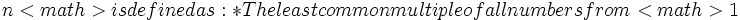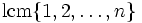Lcm of all numbers so far

(diff) ← Older revision | Latest revision (diff) | Newer revision → (diff)

Definition

Letbe a natural number. The lcm of all numbers so far forto, i.e., as:.

• The product of all primes powersfor whichbut.
• The exponent of the symmetric group of degree.
• It is the exponential of the second Chebyshev function.

Behavior

The ID of the sequence in the Online Encyclopedia of Integer Sequences is A003418

Initial values

The values forare.

Growth

The lcm of all numbers so far has approximately exponential growth in. Moreover, it is not strictly increasing as a function of, and it increases in value only at prime powers. At the prime power, it gets multiplied by.

It is the exponential of the second Chebyshev function. More details on the growth are to be found in the page on the second Chebyshev function.

Relation with other functions

Logarithm

The logarithm of the lcm of all numbers so far is equal to the second Chebyshev function.